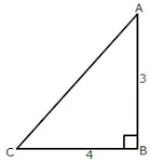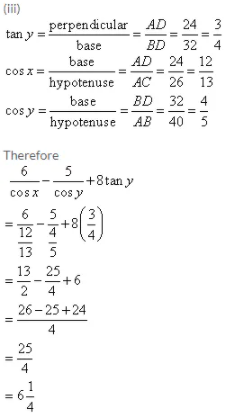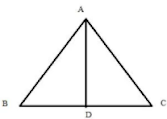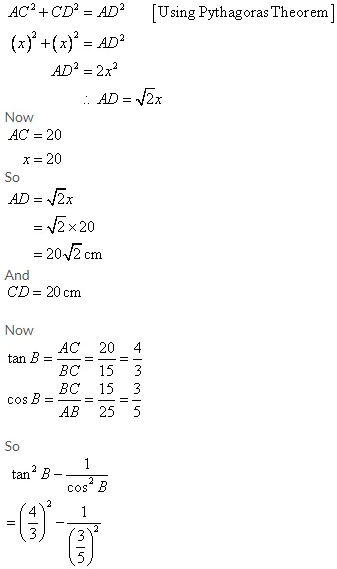# ICSE Solutions for Selina Concise Chapter 22 Trigonometrical Ratios Class 9 Maths

### Exercise 22(A)

1. From the following figure, find the values of :

(i) sin A

(ii) cos A

(iii) cot A

(iv) sec C

(v) cosec C

(vi) tan C.

Given angle ABC = 90°⇒ AC2 = AB + BC (AC is hypotenuse)
⇒ AC2 = 3 + 4
∴ AC2 = 9+16 = 25 and AC = 5
(i) sin A = perpendicular/hypotenuse = BC/AC = 4/5
(ii) cos A = base/hypotenuse = AB/AC = 3/5
(iii) cot A = base/perpendicular = AB/BC = 3/4
(iv) sec C = hypotenuse/base = AC/BC = 5/4
(v) cosec C = hypotenuse/perpendicular = AC/AB = 5/3
(vi) tan C = perpendicular/base = AB/BC = 3/4

2. Form the following figure, find the values of :

(i) cos B

(ii) tan C

(iii) sin2B + cos2B

(iv) sin B. cos C + cos B. sin C

Given angle BAC = 90°⇒ BC = AB + AC (BC is hypotenuse)
⇒ 17= 8+ AC
∴ AC = 289 - 64 = 225 and AC = 15
(i) cos B = base/hypotenuse = AB/BC = 8/17
(ii) tan C = perpendicular/base = AB/AC = 8/15
(iii) sin B = perpendicular/hypotenuse = AC/BC = 15/17
cos B = base/hypotenuse = AB/BC = 8/17
sinB + cosB = (15/17) + (8/17)
= (225 + 64)/289
= 289/289
= 1
(iv) sin B = perpendicular/hypotenuse = AC/BC = 15/17
cos B = base/hypotenuse = AB/BC = 8/17
sin C = perpendicular/hypotenuse = AB/BC = 8/17
cos C = base/hypotenuse = AC/BC = 15/17
sinB. cosC + cosB. sinC = (15/17)×(15/17) + (8/17)×(8/17)
= (225+64)/289
= 289/289
= 1

3. From the following figure, find the values of :

(i) cos A (ii) cosec A

(iii) tan2A – sec2A (iv) sin C

(v) sec C (vi) cot2 C – 1/sin² C

Consider the diagram as

angle ADB = 90° and BDC = 90°
⇒ AB = AD + BD (AB is hypotenuse is △ABD)
⇒ AB = 3 + 4
∴ AB = 9+ 16 = 25 and AB = 5
⇒ BC = BD + DC (BC is hypotenuse in △BDC)
⇒ DC = 12 - 4
∴ DC = 144 - 16 = 128 and DC = 8√2
(i) cos A = base/hypotenuse = AD/AB = 3/5
(ii) cosec A = hypotenuse/perpendicular  = AB/BD = 5/4
(iii) tan A = perpendicular/base = BD/AD = 4/3
sec A = hypotenuse/base = AB/AD = 5/3
tan A - se A = (4/3) - (5/3)
= (16/9) - (25/9)
= -9/9
= -1
(iv) sin C = perpendicular/hypotenuse = BD/BC = 4/12 = 1/3
(v) sec C = hypotenuse/base = BC/DC = 12/8√2 = 3/2√2 = 3√2/4
(vi) cot C = base/perpendicular = DC/BD = 8√2/4 = 2√2
sin C = perpendicular/hypotenuse = BD/BC = 4/12 = 1/3
cotC - 1/sinC = (2√2) - 1/(1/3)
= 8- 9
= -1

4. From the following figure, find the values of :

(i) sin B

(ii) tan C

(iii) sec2 B – tan2

(iv) sin2C + cos2C

5. Given: sin A = 3/5 , find :

(i) tan A

(ii) cos A

Consider the diagram below :

6. From the following figure, find the values of :

(i) sin A

(ii) sec A

(iii) cos2 A + sin2A

Given angle ABC = 90° in the figure

7. Given: cos A = 5/13

Evaluate: (i)( sin A – Cot A )/2 Tan A

(ii) cot A+ (1 / cos A)

Consider the diagram below :

8. Given: sec A =29/11, evaluate: sin A – 1/ Tan A

Consider the diagram below :

9. Given: tan A =4/3, find : cosecA/(cot A-sec A)

Consider the diagram below :

10. Given: 4 cot A = 3 find;

(i) sin A

(ii) sec A

(iii) cosec2 A – cot2A.

Consider the diagram below :

11. Given: cos A = 0.6; find all other trigonometrical ratios for angle A.

Consider the diagram below :

12. In a right-angled triangle, it is given that A is an acute angle and tan A =. 5/12

find the value of :

(i) cos A

(ii) sin A

(iii) (cos A + sin A)/(cos A – Sin A)

Consider the diagram below :

13. Given: sin θ = p/q

Find cos θ + sin θ in terms of p and q.

Consider the diagram below :

14. If cos A =1/2 and sin B = 1/√2  , find the value of : .

tan A–tan B/(1+tan A tan B)

Are angles A and B from the same triangle? Explain.

Consider the diagram below :

15. If 5 cot θ = 12, find the value of : Cosec θ + sec θ

Consider the diagram below :

16. If tan x = 1 1/3, find the value of : 4 sin2x – 3 cos2x + 2

Consider the diagram below :

17. If cosec θ= √5, find the value of:

(i) 2 – sin2θ- cos2θ

(ii) 2 + (1+sin2θ) - (cos2θ/sin2θ)

Consider the diagram below :

18. If sec A =√2 , find the value of : (3cos2 A + 5 tan2 A)/(4tan4 A - sin2 A)

Consider the diagram below :

19. If cot = 1; find the value of: 5 tan2θ + 2 sin2θ – 3

Consider the diagram below :

20. In the following figure:AD⟂BC, AC = 26 CD = 10, BC = 42,

∠DAC = x and B = y.

Find the value of :

(i) cot x

(ii) 1/sin2 y - 1/tan2 y

(iii) 6/cos x - 5/cos y + 8 tan y.

Given,

∠DAC = 90° and ∠ADB = 90° in the figure### Exercise 22(B)

1. From the following figure, find:

(i) y

(ii) sin xo

(iii) (sec xo – tan xo) (sec xo + tan xo)

Consider the given figure

2. Use the given figure to find:(i) sin xo

(ii) cos yo

(iii) 3 tan xo – 2 sin yo + 4 cos yo.

Since, the triangle is a right angled triangle, so using Pythagorean Theorem

3. In the diagram, given below, triangle ABC is right-angled at B and BD is perpendicular to AC.Find:

(i) cos ∠DBC

(ii) cot ∠DBA

Since the triangle is a right angled triangle.

So using Pythagorean Theorem,

AC2 = 52 + 122

⇒ AC2 = 25 + 144

⇒ AC2 = 169

⇒ AC = 13

In △CBD and △CBA, the C is common to both the triangles, ∠CDB = ∠CBA = 90°, so therefore ∠CBD = ∠CAB.

Therefore, △CBD and △CBA are similar triangles according to AAA Rule.

4. In the given figure, triangle ABC is right-angled at B. D is the foot of the perpendicular from B to AC. Given that BC = 3 cm and AB = 4 cm. find:

(i) tan DBC

(ii) sin DBA

Consider the given figure

5. In triangle ABC, AB = AC = 15 cm and BC = 18 cm, find cos ABC.

Consider the figure belowIn the isosceles △ABC, AB = AC = 15 cm and BC = 18cm the perpendicular drawn from angle A to the side BC divides the side BC into two equal parts BD = DC = 9 cm

6. In the figure given below, ABC is an isosceles triangle with BC = 8 cm and AB = AC = 5 cm. Find:

(i) sin B

(ii) tan C

(iii) sin2 B + cos2

(iv) tan C – cot B

Consider the figure belowIn the isosceles △ABC, AB = AC = 5cm and BC = 8cm the perpendicular drawn from angle A to the side BC divides the side BC into two equal parts  BD = DC = 4cm

7. In triangle ABC; ABC = 90o, CAB = xo, tan xo = and BC = 15 cm. Find the measures of AB and AC.

Consider the figure= 25 cm

8. Using the measurements given in the following figure:

(i) Find the value of sin and tan.

(ii) Write an expression for AD in terms of

Consider the figureA perpendicular is drawn from D to the side AB at point E which makes BCDE is a rectangle.
Now in right angled triangle BCD using Pythagorean Theorem

9. In the given figure;

BC = 15 cm and sin B =.

(i) Calculate the measure of AB and AC.

(ii) Now, if tan ADC = 1; calculate the measures of CD and AD.

Also, show that: tan2B – 1/cos² B = -1

Given
Sin B = 4/5
i.e.   perpendicular /hypotenuse  = AC/AB = 4/5
Therefore if length of perpendicular  = 4x, length of hypotenuse = 5x
Since,10. If sin A + cosec A = 2;

Find the value of sin2 A + cosec2 A.

sin A + cosec A = 2
Squaring both sides

11. If tan A + cot A = 5;

Find the value of tan2 A + cot2 A.

tan A + cot A = 5
Squaring both sides

12. Given: 4 sin = 3 cos ; find the value of:

(i) sin

(ii) cos

(iii) cot2 – cosec2.

(iv) 4 cos2– 3 sin2+ 2

13. Given : 17 cos = 15;

Find the value of : tan + 2 sec.

Consider the diagram below :

14. Given : 5 cos A – 12 sin A = 0; evaluate :
(sin A + cos A)/(2cos A - sin A)

5 cos A - 12 sin A = 0
⇒ 5 cos A = 12 sin A

15. In the given figure; C = 90o and D is mid-point of AC. Find

(i) tan CAB/tan CDB

(ii) tan ABC/tan CDB

Since D is mid - point of AC so AC = 2DC

16. If 3 cos A = 4 sin A, find the value of :

(i) cos A

(ii) 3 – cot2 A + cosec2A.

Consider the diagram below :

17. In triangle ABC, B = 90o and tan A = 0.75. If AC = 30 cm, find the lengths of AB and BC.

Consider the figure

18. In rhombus ABCD, diagonals AC and BD intersect each other at point O.

If cosine of angle CAB is 0.6 and OB = 8 cm, find the lengths of the the side and the diagonals of the rhombus.

Consider the figure

19. In triangle ABC, AB = AC = 15 cm and BC = 18 cm. Find:

(i) cos B

(ii) sin C

(iii) tan2 B – sec2 B + 2

Consider the figure below

20. In triangle ABC, AD is perpendicular to BC. sin B = 0.8, BD = 9 cm and tan C = 1. Find the length of AB, AD, AC and DC.

Consider the figure below

21. Given q tan A = p, find the value of :
(p sinA - q cosA)/(p sinA + q cosA)

22. If sin A = cos A, find the value of 2 tan2A – 2 sec2 A + 5.

Consider the figure

23. In rectangle ABCD, diagonal BD = 26 cm and cotangent of angle ABD = 1.5. Find the area and the perimeter of the rectangle ABCD.

Consider the diagram

24. If 2 sin x = , evaluate.

(i) 4 sin3 x – 3 sin x.

(ii) 3 cos x – 4 cos3 x.

Consider the figure

25. If sin A = √3/2 and cos B = √3/2, find the value of :
(tan A - tan B)/(1+tanA . tan B) .

Consider the diagram below :

26. Use the information given in the following figure to evaluate:

10/sin x + 6/sin y -  6 cot y

Consider the given diagram as

27. If sec A = √2 , find: (3 cot2 A + 2 sin2 A)/(tan2 A - cos2 A) .

Consider the figure

28. If 5 cosθ = 3, evaluate : (cosecθ - cotθ)/(cosecθ + cotθ) .

29. If cosec A + sin A = 5,1/5 find the value of cosec2A + sin2A.